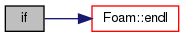The OpenFOAM FoundationlogSummary.H File Reference

Go to the source code of this file.

## Functions

if (Pstream::master())

## Variables

const scalar meanT = T.weightedAverage(mesh.V()).value()

const scalar meanup

## ◆ if()

 if ( Pstream::master() )

Definition at line 7 of file logSummary.H.

References Foam::endl(), Foam::Info, logSummaryFile, runTime, and Foam::tab.

Here is the call graph for this function:## ◆ meanT

 const scalar meanT = T.weightedAverage(mesh.V()).value()

Definition at line 3 of file logSummary.H.

## ◆ meanup

 const scalar meanup
Initial value:
=
(sqrt((2.0/3.0)*turbulence->k()))().weightedAverage(mesh.V()).value()
dimensionedScalar sqrt(const dimensionedScalar &ds)
dynamicFvMesh & mesh
Info<< "Reading field U\"<< endl;volVectorField U(IOobject("U", runTime.timeName(), mesh, IOobject::MUST_READ, IOobject::AUTO_WRITE), mesh);volScalarField rho(IOobject("rho", runTime.timeName(), mesh, IOobject::NO_READ, IOobject::AUTO_WRITE), thermo.rho());volVectorField rhoU(IOobject("rhoU", runTime.timeName(), mesh, IOobject::NO_READ, IOobject::NO_WRITE), rho *U);volScalarField rhoE(IOobject("rhoE", runTime.timeName(), mesh, IOobject::NO_READ, IOobject::NO_WRITE), rho *(e+0.5 *magSqr(U)));surfaceScalarField pos(IOobject("pos", runTime.timeName(), mesh), mesh, dimensionedScalar(dimless, 1.0));surfaceScalarField neg(IOobject("neg", runTime.timeName(), mesh), mesh, dimensionedScalar(dimless, -1.0));surfaceScalarField phi("phi", fvc::flux(rhoU));Info<< "Creating turbulence model\"<< endl;autoPtr< compressible::momentumTransportModel > turbulence(compressible::momentumTransportModel::New(rho, U, phi, thermo))
Definition: createFields.H:94

Definition at line 4 of file logSummary.H.